## Dynamics

The study of dynamics goes beyond the relationships between the variables of motion as illuminated in kinematics to the cause of motion, which is force.

## Newton's laws of motion

Newton's first law of motion, also called the law on inertia, states that an object continues in its state of rest or of uniform motion unless compelled to change that state by an external force. The law appears to contain two separate statements. The first statement—that a state of rest will continue unless a force is applied—seems intuitively correct. The second statement—that an object will continue with a constant velocity unless compelled to change by an impressed force—seems contrary to common experience. It is important to realize that objects observed to slow down are being compelled to change by a frictional force. Friction is a retarding force that is ever present in our everyday world. For the ideal—the absence of outside forces acting on the object, as described by the law—friction must be eliminated. The value of the law is the introduction of the concept of force as a push or pull that causes a body to change its state of motion.

Newton's second law of motion states that if a net force acts on an object, it will cause an acceleration of that object. The law addresses the cause and effect relationship between force and motion commonly stated as F = m a, where m is the proportionality constant (mass). Force is measured in SI units of newtons, abbreviated N.

Newton's third law of motion states that for every action there is an equal and opposite reaction. Therefore, if one object exerts a force on a second object, the second exerts an equal and oppositely directed force on the first one.

## Mass and weight

Mass and weight are distinctly different physical quantities, a fact that cannot be emphasized too strongly. Mass is the property that lends an object a reluctance to change its state of motion. Mass is the measure of the amount of matter in an object. Masses are compared on an equal‐arm balance. If a loaded two‐pan balance is level on earth, it will be level in a different gravitational field, as for example, on the moon. Thus, mass is an invariant quantity; it is measured in units of kilograms. A mass of 1 kilogram will experience an acceleration of 1 m/s 2 under the action of a force of 1 newton.

The force that the earth exerts on an object of specific mass is called the object's weight on earth. Weight is a force measured in units of newtons and is a vector quantity. The expression for weight is W = m g, where g is the acceleration due to gravity. A spring scale translates the force of attraction between an object and the earth into a reading of weight. In contrast to a measurement of mass, weight is not an invariant. An object on a spring scale on earth would not weigh the same on the moon because the pull of gravity on the object differs in the two locations.

## Force diagrams

To better understand the relationship between force and acceleration in a particular case, it is helpful to use a force diagram, also called a free‐body diagram. An object that is not moving is said to be in a static equilibrium. An example is a weight hanging by two ropes from the ceiling (see Figure ). To analyze this problem, consider the forces acting on the knot joining the ropes. Then,

1. Make the force diagram.

2. Find the components of forces not directed along the coordinate axes and write the force equation for each axis.

3. Solve the simultaneous equations for the tensions.Figure 1

Force diagram of a suspended weight.

The following examples illustrate these procedures. (All of the following vector diagrams are drawn to scale.)

Example 1: What is the tension in each rope in Figure ?

1. Make the force diagram.

• The tension in the lower rope attached to the mass must be mg directed downward; therefore, T 3 = − mg.

2. Find the components of forces not directed along the coordinate axes and write the force equation for each axis.

• Components of the forces in the x direction are• Components of the forces in the y direction are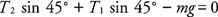3. Solve the simultaneous equations for the tensions.

Solution:To minimize computation errors, show the components on a separate force diagram as shown in Figure .

Example 2: Now, see if you can make the free‐body diagram and set up the force equations for a pail on the end of a rope that is accelerating upward. Find the tension in the rope and the acceleration of the pail. See Figure (a).Figure 2

Force diagram of a bucket being lifted.

The two forces acting on the pail are the tension of the rope ( T) and weight ( W = mg). By Newton's second law:

Solution: F net = Tmg = ma

Example 3: Next, try to set up the equations for a two‐body system of unequal masses attached by a rope over a frictionless pulley (see Figure ). A diagram must be made for each of the two objects of mass ( m 1) and ( m 2). Find the acceleration of the system and the tension in the rope.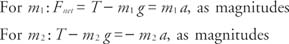Figure 3

Force diagram of two masses hung over a pulley.

The second equation has a negative acceleration because m 2 is descending. Because the objects are connected by a rope that does not expand, the tensions and accelerations are the same for each mass. By algebraic manipulation, the equations may be simultaneously solved for the following results:

Solution:Example 4: In Figure , one object sits on a frictionless surface, and the other object hangs off the edge of the table over a pulley. Make the free‐body diagram and write the force equations to find the acceleration and tension.Figure 4

Same as Figure 3 with one mass on a frictionless table.

In the vertical direction, the forces on m are the weight downward and the normal force ( N) upward due to the surface, which is equal and opposite to the weight. Because there is no acceleration of m 1 in this direction, the net vertical force is zero. A horizontal force of the tension in the rope accelerates the mass to the right. Write the force equations separately for x and y directions for m 1. The forces on the second mass are the same as those in the last example. For m 1 in the x direction: T = m 1 a; in the y direction: Nm 1 g = 0. For m 2: Tm 2 g = − m 2 a. Combining the two equations gives the relationships.

Solution:Even more complicated problems can be separated into manageable parts to allow solution by using these problem‐solving methods.

Example 5: Consider a mass ( m 1) on an inclined plane attached to a mass ( m 2) over a pulley as in Figure (a). Both the plane and pulley are frictionless. Set up the problem to find the acceleration.Figure 5

Now the table in Figure 4 is tilted at an angle.

In this case, the forces on m 1 must be resolved in components along the x and y axes. The coordinate system with the x axis parallel to the surface of the plane is selected so that only one force, the weight of m 1, needs to be converted into component form. The normal force is always perpendicular to the plane and in this case, therefore, is opposed only be the component of weight that is also perpendicular to the plane's surface. Note that the angle between the y axis and the weight ( m 1 g) is the angle of inclination of the plane, which can be proven by geometry. The coordinate system for m 2 has the same orientation as in the previous example. Assume m 2 > m 1. Then, for m 1 in its y direction: Nm 1 g cos θ = 0; in its x direction: Tm 1 g sin θ = m 1 a. For m 2: Tm 2 g = − m 2 a. The acceleration is then

Solution:and tension is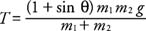To analyze a physical situation by the use of free‐body diagrams, use the following steps:

1. Make a free‐body diagram for each object. If one object is sitting on a surface, be sure to include the normal force.

2. Resolve the forces that are not directed along the x and y axes into components along a preferred coordinate system. For inclined planes, use a coordinate axis with the x axis parallel to the surface of the plane. Put the components on a separate diagram; that is, do not put the force and its components on the same diagram because this combination might complicate the following steps.

3. Write out the force equation for each mass along each axis, noting the correct sign for the acceleration of the body.

4. Solve the equations simultaneously to find the desired value(s).

## Friction

Friction is the force opposing the motion of one body sliding or rolling over the surface of second object. Several aspects of friction are important at low velocities:

• The direction of the force of friction is opposite the direction of motion.
• The frictional force is proportional to the perpendicular (normal) force between the two surfaces in contact.
• The frictional force is nearly independent of the area of contact between the two objects.
• The magnitude of the frictional force depends on the materials composing the two objects in contact.

Static friction is the force of friction when there is no relative motion between two objects in contact, such as a block sitting on an inclined plane. The magnitude of the frictional force is F s ≤ μ s N, where N is the magnitude of the normal force, and the coefficient of friction (μ s) is the dimensionless proportionality constant. The coefficient of friction may be approximately .2 for normal lubricated surfaces and close to 1 for glass sliding on glass. This equation sets the upper limit for the static frictional force. If a greater external force is applied, the situation will no longer be static, and the object will begin to move.

Kinetic friction is the force of friction when there is relative motion between two objects in contact. The magnitude of the friction force in this case is F k ≤ μ k N, where N is the magnitude of the normal force and μ k is the coefficient of kinetic friction. Note that μ k is not strictly a constant, but this empirical rule is a good approximation for finding frictional forces. Values given for the coefficients of static and kinetic friction do vary with speed and surface conditions so that it is not necessarily true that static friction exceeds sliding friction.

The following problem highlights the differences between static and kinetic friction.

Example 6: A block sits on an inclined plane. What is the maximum angle that which the block remains at rest? First, draw the free‐body diagrams and then write out the force equation for each direction of the coordinate system (see Figure ).Figure 6

A block on an inclined plane, with friction.

Suppose the surface is tilted to θ, at which the block just begins to move. Then, the force down the plane must be equal to the maximum force of static friction; thus f friction = μ s N. Therefore, f friction = mg sin θ = μ s N = μ s ( mg cos θ) and solving for the coefficient of friction:

Solution: μ s = tan θ

At a greater angle of tilt, the object accelerates down the surface, and the force of friction is f k = μ k N.

Example 7: If the surfaces in Figures and were not frictionless, the frictional force parallel to the surface and opposite the direction of motion must be included in the analysis. Pulling a block along a horizontal surface at a constant speed (zero acceleration) is an example of a problem involving friction, and such a block is analyzed in Figure .Figure 7

Pulling a block on a plane with friction.

In the x direction, T cos θ − f = 0, where f is the friction force and T is the tension in the rope. In the y direction, N + T sin θ − mg = 0; also, f = μ k N.

Solve the y direction equation for N, substitute the expression into the friction force equation, and then substitute friction into the first equation to obtain the following: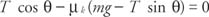Solution: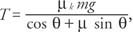solving for T.

## Centripetal force

When an object rotates in a circle, a force must be directed to the center of the circle to maintain the motion; otherwise, the object will take off tangent to the path. This constraining force is called a centripetal force, meaning center‐seeking. In the example of a mass rotating in a horizontal circle at the end of the string, the centripetal force is provided by the tension in the string. In the case of orbiting satellites, gravity provides the center‐seeking force. From the definition of force F = m a and the expressions for circular accelerations, the following equations are obtained: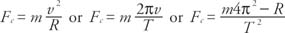If an object moves in a circle, the net force is a centripetal force. One such example is the conic pendulum, a mass on the end of a string that rotates in a horizontal circle (see Figure ).Figure 8

A conic pendulum

In the y direction, T cos θ − mg = 0. In the x direction (or the radial direction), T sin θ = mv 2/ R, where R is the radius of circular path. Dividing the second equation by the first equation and solving for v yields the following:## Universal gravitation

Newton's law of universal gravitation states that every mass in the universe attracts every other mass with a gravitational force that is directly proportional to the product of their masses ( m 1, m 2) and inversely proportional to the square of distance (r) between them. In mathematical form, F = ( Gm 1 m 2/ r 2), where G is the universal gravitational constant. In the metric system, the accepted value of G is G = 6.673 × 10 −11 ( Nm 1/ kg 2).

Kepler found three empirical laws regarding the motion of satellites that Newton later showed from his law of universal gravitation. These are Kepler's laws of planetary motion:

• The law of orbits: All planets move in elliptical orbits with the sun at one focus.
• The law of areas: A line joining a planet and the sun sweeps out equal areas in equal time.
• The law of periods: The square of the period (T) of any planet is proportional to the cube of the semi‐major axis ( r) of its orbit, or T 2 = (4μ 2/ GM) r 3, where M is the mass of the planet.

## Momentum and impulse

According to Newton's second law, a mass experiencing a net average force ( F) for a time interval Δ t will undergo an average acceleration ( F= m a). The product of the average force acting on the body and time of contact is defined as impulse. Because acceleration is change in velocity, the relationships between these variables are expressed as the Impulse = F (Δ t) = mv fmv i, where v i is the initial velocity and v f is the velocity after the force is no longer in contact with the body. Impulse is measured in units of newton‐seconds, or more simply, N‐s.

When applying the impulse equation, be sure to calculate the vector change in velocity—for example, consider a mass of 10 kg acted on by a force that changes its velocity from −8 m/s to 3 m/s . This force imparted an impulse of (10 kg)[3 − (−8) m/s] = (10 kg)(11 m/s) = 110 N‐s.

The right side of the impulse equation is the change in the linear momentum of the object. The definition of linear momentum is p = m v. Linear momentum is measured in units of kilogram meters/second or, in abbreviated form, kg m/s. Newton originally stated his second law by saying that the rate of change of momentum with time is proportional to the impressed force and is in the same direction; thus, F = Δ ( mv)/Δ t or F = Δp/Δ t.

## Conservation of momentum

An extremely important fundamental principle in physics is the law of conservation of momentum. The law states that if there is no external force acting on a system, the total momentum remains a constant, which provides a powerful way to analyze interactions between systems of objects. For example, if a rolling ball on a frictionless surface collides with another ball, the total momentum before and after the collision is the same. An interaction, therefore, can be examined without knowing the forces involved and the length of interaction time, which might be difficult to measure.

First, consider a head‐on collision (so it is not necessary to utilize two‐dimensional vectors, that is, consider only straight line motion). Imagine a mass ( m 1) with velocity ( v 1) hitting a mass ( m 2) that is initially at rest. The momentums are the following: before the collision, m 1 v 1, and after the collision, m 1 v 1 + m 2 v 2, where the primes indicate velocities after the interaction. From the law of conservation of momentum, the two expressions may be set equal to each other. Consider the special case where the two masses are equal on frictionless surface and stick together after the collision (have the same primed velocity). Then, total momentum before the collision equals total momentum after the collision, mv 1 = mv′ + mv′; therefore, v′ = (1/2)(v 1), or the final velocity is one‐half the original velocity because the effective mass has doubled.

Another way to state the law of conservation of momentum is that the change in momentum of the two objects must be equal and opposite. For example, two ice skaters are at rest in the center of frictionless ice (possible at least in the imagination). Let one have a relatively small mass (m) and the other a larger mass (M). Because they begin at rest, the initial momentum is zero. They then push apart in opposite directions. The total momentum must remain zero.

According to the law of conservation of momentum, Δp m = −Δp m or mv′ − 0 = −( MV′−0); therefore, if the large mass (M) is three times the smaller mass (m), v′ = −3V′, where v′ is the velocity of the small mass after the collision and V′ is the velocity of the large mass after the collision. The negative sign indicates velocities in opposite directions.

This same analysis holds for a person standing on frictionless ice who throws an object; it even holds for a rocket going to the moon. The ice skater throwing a glove attains equal momentum in the direction opposite to that of the thrown object. This basic principle is the same for a rocket accelerating in space. Spacecrafts utilize the law of conservation of momentum in getting an additional push from discharged rocket stages as well as from fuel. In particular, the Apollo space capsule returning from the moon was only a small percent of the total mass initially sent upward from the launch pad; therefore, acceleration of a rocket can be caused by either a change in velocity, by a change in its mass, or by changes in both velocity and mass (see Figure ). Thus, the expression of Newton's second law of motion, stated in terms of the change of momentum, is broader than the expression given only in terms of mass and acceleration.Figure 9

A rocket ship gains momentum.

If two objects strike with a glancing blow, the motion will be two‐dimensional. For example, one ball ( m 1) with an initial velocity hits a second ball ( m 2), which is initially at rest. Figures and depict this situation with the first ball initially moving up from the bottom of the page. For the sake of simplicity, allow the two masses to be equal.Figure 10

Two balls striking a glancing blow.Figure 11

(a) Total momentum is conserved. (b) Equal and opposite momentum changes of the two balls.

The momentum vectors can be added to show the law of conservation of momentum. The vector addition in Figure (a) shows that the total of the two momentum vectors, p1′ and p2′, after the collision are equal to the total momentum before the collision. (Because only m 1 was moving, there was only one initial momentum vector, p1.) Figure (b) also shows the alternate method of using the law of conservation of momentum, that the change (difference) in momentum of m 1 is equal and opposite to that of m 2.

If the two masses are not equal, the velocity vectors must be adjusted so that the vectors represent momentum. For example, if one mass is three times the other, the velocity vectors of the larger mass must be lengthened by a factor of three before using the law of conservation of momentum.